Go to   Fun Math Content Table   Pursuit Curves Home

# Topics from Pursuit Curves

### How to catch up a boat.--Apollonius circle

List of animations posted on this page.(Click the text to watch animation.)
Case (a) Facing each other
Case (b) Chasing behind on a line
Case (c) General case

Suppose a ship leaves point B and steams in a fixed direction at a constant speed. A second ship,leaving point A at the same time, can go K times as fast as the first ship. Assuming a plane ocean,what course should the first ship steer in order to intercept the slow ship as quickly as possible ?******* Apollo_circle_00.dwg ********

Let us begin with two very simple hypothetical cases when both ships are on the same line.

#### Case (a) Facing each other

It is easy to guess that two ships will meet at Point C,
which divides line AB internally to the K:1 ratio,i.e AC:CB = K : 1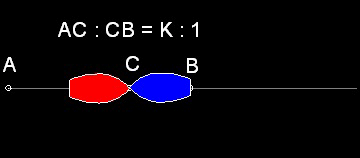******* Apollo_circle_01.dwg ******** ******* Apollo_circle_01A.dwg *******

You can see the process in animation.

#### Case (b) Chasing on the same straight line

In this case the Chaser will catch up the slower ship at Point D,
which divides line AB externally to the K:1 ratio,i.e AD:BD = K:1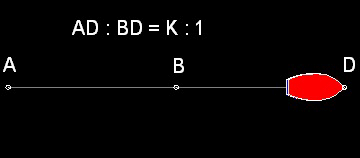******* Apollo_circle_02.dwg ******** ******** Apollo_circle_02A.dwg ********

You can see the process in animation.

#### Case (c) More general case

What direction the boat "A" should take when the slower boat is moving in the direction
not colinear with line AB as shown below ?******* Apollo_circle_00.dwg ********

Suppose the point of interception is P. Then this point P has to satisfy the following condition:

`            AP:BP = K : 1  `
For example let the value of K be set equal to 2. Draw a circle with its center at B and radius R1. Then draw a circle with its center at A and radius K times R1. Locate the intersection points. Do this process a few times. The result is as follows: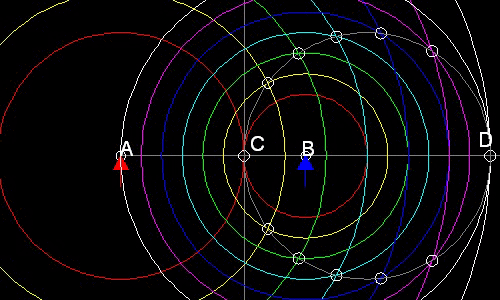You will notice the following:
1. Points C & D are the cases when a pair of circles are tangent.(red and white circles.)
2. All the intersection points are on the circle with CD as a diameter.(yellow,cyan,blue,& magenta circles)
To draw multiple Apollonius circles
from command line, type    test_circle
red_arrow.dwg & blue_arrow.dwg are required.
******************* Apollo_03.dwg **********************

The Geeek mathematician Apollonius discovered that a circle may be defined in a way quite different from the usual "all points equidistant from a fixed point." Apollonius's defintion states that if a point moves in such a way that its distance from one fixed point is always a constant multiple of its distance from another fixed point, then its path is a circle.

It is now clear what the chaser has to do in order to catch up with a slower boat.
1. Based on the value of K, find out the position C & D.
2. Draw a circle with CD as a diameter.
3. Find out the point P on the circle where the slower ship is heading for.
4. Steer the boat to the direction of Point P.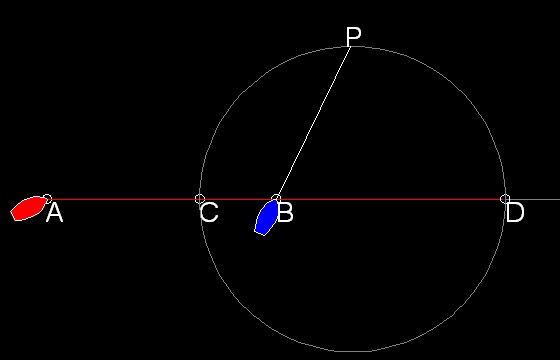You can see the process in animation.

To create this drawing and animation:
To see case (a),(b),& (c)

from command line, type    pursuit_test
Case (a), (b) & (c) runs continuously.
Note* : This program requires red_boat.dwg & blue_boat.dwg .

********************** Apollo_1c_0.dwg ************************

After you run the general case, the result will look like this.#### What is special about points C & D ?

Draw a line BF from point B perpendicular to DP.
The extensions of AP and BF meet at point E.

If angles APC, CPB, BPD, and DPE are measured, we will find out that points C & D bisect both internal and external angles at apex P of triangle APB.

******************* pursuit_Apollonius_01.dwg ********************

More on the Apollonius Circle:

Letting the mid-point between A & B is O, and AO = BO = r.
1. Then OC = r*(K-1)/(K+1)

OD = r* (K+1)/(K-1)

2. The diameter of the Apollonius circle is r * 4K/(K2 -1)
3. The distance of the center of the circle from point "O" is r*(K2 + 1)/(K2 - 1)

#### Discussion on Geometric Proof

The discussion above are based on the following Theorems proved by Apollonius.

1. If AB is divided harmonically by C and D,then CD is also harmonically divided by A and B

2. The bisector of any angle of a trianlge divides the opposite side into parts proportional to the adjacent sides.

AC/CB = AD/BD = AP/BP = K

In the following drawing, CP and DP are the angle bisectors, and

CP is parallel to BE, and BG is parallel to DP.

#### Summary of proof:

In Triangle ABE, since CP is parallel to BE, AC/CB = AP/PE = AP/BP

In Triangle ADP,since BG is parallel to DP, AD/BD = AP/GP = AP/BP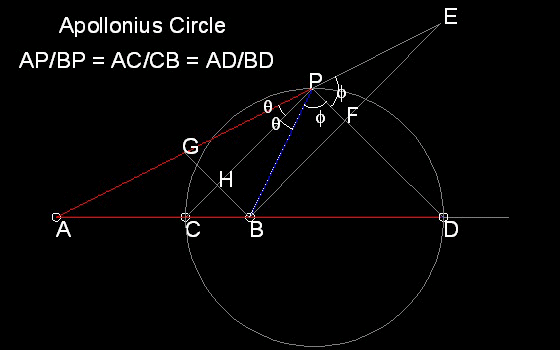To create this drawing and animation:
To see case (a),(b),& (c)

from command line, type    pursuit_test
Case (a), (b) & (c) runs continuously.
Note* : This program requires red_boat.dwg & blue_boat.dwg .
****************** Apollo_circle_03.dwg *********************

Go to   Fun Math Content Table   Pursuit Curves Home

All comments/suggestions should be sent to Takaya Iwamoto

Last Updated Aug-10th, 2006